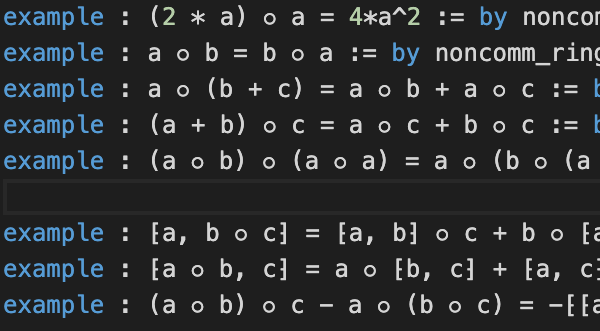## The Mathlib formalisation project needs your help

### A serious effort to formalise modern mathematics

Mathlib is a digital library of mathematics written in Lean that:
• is formally verifiable by computer,
• is growing rapidly, currently about 350k lines of code,
• uses continuous integration and open source workflows,
• is monolithic, leveraging the unity of mathematics,
• tends to work at approximately the Bourbaki level of generality.
The library is supported by a vibrant and welcoming community and includes resources such as:
An ideal achievement for Mathlib is to enable a mainstream mathematician to formalise their work with only a modest incremental effort. A metric for this might be the proportion of recent papers appearing in Annals of Mathematics (or Inventiones or Crelle or JDG or ...) that have been formalised. Note that this metric is already non-zero!

Mathlib is by no means the only library making a serious effort to formalise mathematics but I believe it is by far the most promising. I also believe that a small number of frontiers in mathematics have reached such a level of complexity that we need tools beyond traditional peer review to be sure of correctness. I am optimistic about the future of Mathlib but I believe that even in a suboptimal scenario where it reaches a natural limit (perhaps where the complexity of formalisation begins to dominate the complexity of the ideas being formalised) it will help us learn how to build the tools we will eventually need.

### An example: Lie algebras

After noticing an open issue calling for help I started working on the formalisation of Lie algebras. I decided that an achieveable goal for which I would aim would be to be able to state the classification theorem for finite-dimensional semisimple Lie algebras over an algebraically closed field of characteristic zero. With help from others, this is now only a stone's throw away. The following working Lean code shows that we need only the definitions of the exceptional Lie algebras in order to be able to state the classification theorem for the simple Lie algebras over the complex numbers:
      
import data.complex.basic
import algebra.classical_lie_algebras

open_locale classical
open lie_algebra lie_algebra.special_linear lie_algebra.orthogonal lie_algebra.symplectic
notation L₁ ≅ L₂ := nonempty (L₁ ≃ₗ⁅ℂ⁆ L₂)

def g₂ : Type* := sorry
instance : lie_ring g₂ := sorry
instance : lie_algebra ℂ g₂ := sorry

def f₄ : Type* := sorry
instance : lie_ring f₄ := sorry
instance : lie_algebra ℂ f₄ := sorry

def e₆ : Type* := sorry
instance : lie_ring e₆ := sorry
instance : lie_algebra ℂ e₆ := sorry

def e₇ : Type* := sorry
instance : lie_ring e₇ := sorry
instance : lie_algebra ℂ e₇ := sorry

def e₈ : Type* := sorry
instance : lie_ring e₈ := sorry
instance : lie_algebra ℂ e₈ := sorry

variables (L : Type*) [lie_ring L] [lie_algebra ℂ L]

def is_exceptional := (L ≅ g₂) ∨ (L ≅ f₄) ∨ (L ≅ e₆) ∨ (L ≅ e₇) ∨ (L ≅ e₈)

def is_classical (n) [fintype n] := (L ≅ sl n ℂ) ∨ (L ≅ so n ℂ) ∨ (L ≅ sp n ℂ)

theorem simple_classification [finite_dimensional ℂ L] [is_simple ℂ L] :
is_exceptional L ∨ (∃ n : ℕ, is_classical L (fin n)) :=
sorry


Direct sums of Lie algebras have already been defined so as soon as we drop in a definition for completely reducible Lie modules, we will be able to state the classification in the semisimple case.

The pace of progress is picking up too. For example in just the past week:

• definitions of the classical Lie algebras were completed,
• the construction of tensor algebras was completed (this will allow construction of the universal enveloping algebra),
• work on derivations has begun (after a little generalisation this will permit construction of the exceptional Lie algebras).
And this was not a coordinated effort: three different people had three different aims. The classical Lie algebras were defined in order to get to the statement of the classification theorem, the tensor algebra was constructed in order to define Clifford algebras (including the exterior algebra) and derivations are being developed in order to define the Lie algebra structure on smooth vector fields. And this is just one corner of Mathlib!

### A world of low-hanging fruit

There are easily thousands of hours of work still needed in order to formalise many easy mathematical concepts. If you believe in the future of formalisation, this means that there is abundant easy work of real value to mathematics that needs doing. Pick your favourite subject (or look here for inspiration) and come and join in the fun.

### Notes to my future self

Much as I enjoy contributing to Mathlib, I must divert my attention elsewhere for the next few months. The following list of missing definitions and results is a note to my future self or to anyone else who feels like contributing to the Lie algebra corner of Mathlib:
• the category of Lie algebras,
• the lattice structure on Lie submodules,
• solvable, nilpotent, semisimple Lie algebras,
• the Killing form, Cartan's criterion for semisimplicity,
• Cartan subalgebras,
• the universal enveloping algebra,
• root systems,
• general non-associative algebras, especially composition algebras.
I think root systems and composition algebras might be especially fun to work on.

The theory of general non-associative algebras is there because there are convenient ways to define the exceptional Lie algebras using the octonions. Moreover, given a vector space, its endomorphisms form a boring Lie algebra but if the vector space carries either:

• a bilinear form, we can look at the Lie subalgebra of endomorphisms that are skew-adjoint, or
• a possibly-non-associative multiplication, we can look at the Lie subalgebra of endomorphisms that are a derivation.
Composition algebras have both a bilinear form and a compatible multiplication making them a doubly-good source of Lie algebras (after polarising compatibility relationship $\|ab\|^2 = \|a\|^2\|b\|^2$, many things drop out).